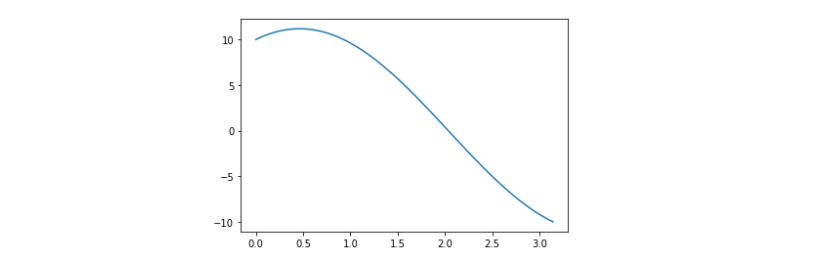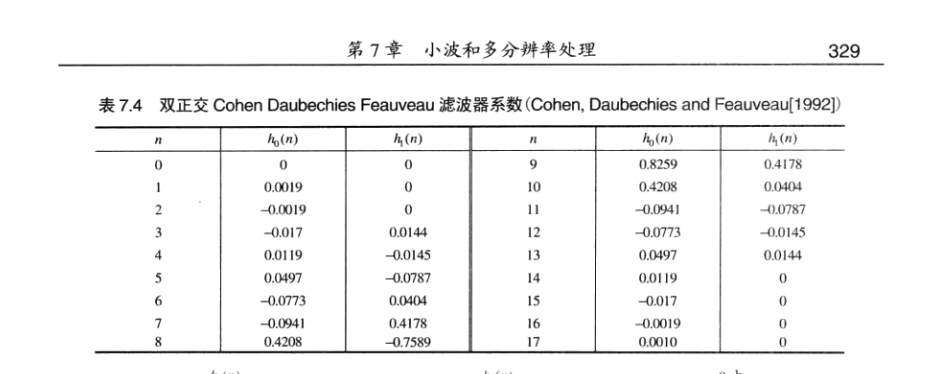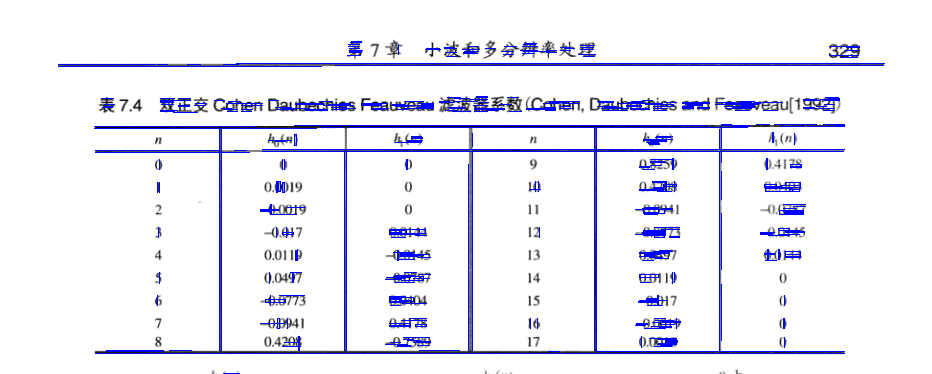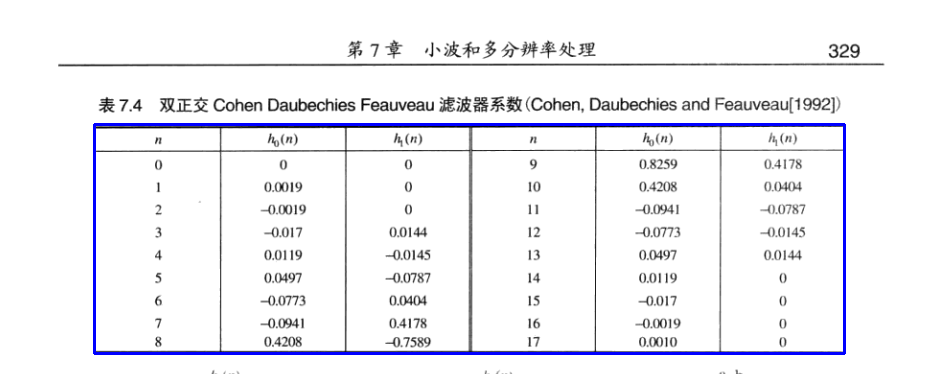Fenrier Lab

# 如何检测出图片中的表格

$r = x \cos \theta + y \sin \theta$$r_0 = x\cos \theta_0 + y \sin \theta_0$OpenCV 库为我们提供了 HoughLines 和 HoughLinesP 方法可以轻松实现直线检测。但距离我们的目标还差的远，以上图为例，我们使用如下代码检测图片上的直线，并绘制出来

img_canny = cv2.Canny(img, 50, 150)
lines = cv2.HoughLinesP(img_canny, 1, np.pi/180, 10, minLineLength=10, maxLineGap=2)1. 初始化数组 visited，长度等于 Vertex 数量，全部设置为 0，标记所有访问过的节点；
2. 初始化变量 compoent_id = 1，作为连通分量id；
3. 声明一个空栈 stack 用于存储 Vertex id，然后将 0 入栈；
4. 如果 stack 不为空，则循环： a. 将 stack 顶部的 Vertex id 出栈，然后查看邻接矩阵的相应行，如果该行的所有元素都为 0，则说明该 Vertex 是孤立的，将 visited 的相应位置标设为 component_id，然后 component_id 自增 1。如果该行含有非 0 元素，则将该行的所有非 0 位置入栈，并将 visited 的相应位置标设为 component_id，以表示它们属于同一个连通分量； b. 如果此时 stack 为空，则检查 visited 数组是否含有 0 元素，如果有，则将第一个 0 元素位置编号入栈，且 compoenent_id 自增 1。

connect_components(neighbor_matrix):
init: visited, compoent_id, stack = 
loop while stack not empty:
i = stack.pop()
isconnect = neighbor_matrix[i]
if isconnect all 0:
visited[i] = component_id
component_id += 1
for j in position where isconnect != 0:
visited[j] = component_id
if stack is empty:
push the first non-zero vistied index to stack
component_id += 1\begin{aligned} p = (1 - s) p_0 + s p_1\\ q = (1 - t) q_0 + t q_1 \end{aligned}

$r(s, t) = \|p-q\|^2$

\begin{aligned} r(s, t) &= \|p_0 - q_0 + (p_1 - p_0)s - (q_1 - q_0) t\|^2\\ &=\|p_1 - p_0\|^2 s^2 +\|q_1 - q_0\| t^2 -2 (p_1 - p_0)\cdot (q_1 - q_0) st + 2(p_0 - q_0) \cdot (p_1 - p_0)s - 2(p_0 - q_0) \cdot (q_1 - q_0) t + \|p_0 - q_0\|^2\\ &= a s^2 + bt^2 - 2c st + 2ds - 2et + f \end{aligned}

\begin{aligned} a &= \|p_1 - p_0\|^2\\ b &= \|q_1 - q_0\|^2\\ c &= (p_1 - p_0)\cdot (q_1 - q_0)\\ d &= (p_0 - q_0) \cdot (p_1 - p_0)\\ e &= (p_0 - q_0) \cdot (q_1 - q_0)\\ f &= \|p_0 - q_0\|^2 \end{aligned}

$\min_{r, s \in [0, 1]} r(s, t)$

\begin{aligned} &\nabla r = 0\\ \Rightarrow &\left( \frac{\partial r}{\partial s}, \frac{\partial r} {\partial t} \right) =0\\ \Rightarrow & (2as -2ct + 2d, 2bt -2cs -2e) = 0 \end{aligned}

$\hat{s} = -\frac{bd - ce}{ab - c^2}, \hat{t} = \frac{ae - cd} {ab - c^2}$

\begin{aligned} r(0, t) &= bt^2 -2et + f\\ r(1, t) &= b t^2 - (2c+2e) t + a + 2 d + f \\ r(s, 0) &= a s^2 + 2 d s+ f\\ r(s, 1) &= a s^2 + (2d -2c) s+ b - 2 e + f \end{aligned}

\begin{aligned} t_0 &= \frac{e} {b}\\ t_1 &= \frac{c+e} {b}\\ s_0 &= -\frac{d}{a}\\ s_1 &= \frac{c -d} {a} \end{aligned}

$r(0, 0), \quad r(1, 0), \quad r(0, 1), \quad r(1, 1)$

$\|p_1 - p_0\|^2 \|q_1 - q_0\|^2 - ((p_1 - p_0)\cdot (q_1 - q_0))^2 = 0$

[ | ]

$\|\mathbf{a}\|^2 \|\mathbf{b}\| - (\mathbf{a} \cdot \mathbf{b})^2 = \|\mathbf{a} \times \mathbf{b}\|$

$(p_1 - p_0)\times (q_1 - q_0) = 0$